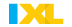•

Math ResourcesLog in to view the textbook, which will be one resource used this year, here:Go to www-k6.thinkcentral.com

Make sure you select:

 State: NEW YORK district: GARDEN CITY SCHOOL DISTRICT, GARDEN CITY 11530 school: STRATFORD AVENUE ELEM SCHOOL, GARDEN CITY 11530 And finally...

Math Skills PracticeMr. Madden's students will be given an account to the XtraMath website!  There, students can strengthen their computation accuracy and fluency!

Click on the link below for games to review the multiplication tables. Scroll down for the games.

The site has many other resources to practice 4th grade math skills.- Practice sample questions on every fourth grade math topic (you are limited to a certain number of questions each day without an account)

Topics of Study for The Current School Year:

As part of the new Common Core curriculum, the students will be expected to understand and explain the concept behind each idea taught, using the appropriate math vocabulary.

Plus go to this site for tons of practice:

I. Number Sense and Operations in Base Ten (addition and subtraction)

-
Here's a fun place value game online!

Lesson- Reading and Writing Multi-Digit Whole NumbersLesson- Rounding Using a Number Line (view strategy video)

Lesson- Comparing Numbers

Lesson- Ordering Numbers

Lesson- Standard Algorithm for Addition (view strategy video)

II.  Reading Time & Elapsed Time

-Interactive Elapsed Time Website

III. Number Sense and Operation in Base Ten (multiplication and division)

A. Multipication

Factors Game and MORE

Lesson - multiplying 2-digit by 2-digit numbers using an array (view strategy video)

Lesson- place value relationships (view strategy video)

Lesson  - multiplication comparisions using algebra Part I (view strategy video)

Lesson - multiplication comparisons using algebra Part II

B. Division:
-practice your division (or any operation) facts

Lesson 1- Meaning of Division (watch video)

Lesson 2- Using Patterns to Divide Mentally (View PowerPoint)

Lesson 3- Circle Division (watch video)

Lesson 4- Partial Quotient Algorithm (watch video)

Lesson 5- Standard Algorithm

Lesson 6- Interpreting Remainders (watch video)

Lesson 7- Area Model and Division (watch video)

Lesson 8- Multi-Step Problems (with Area and Perimeter) (watch video)

IV. Geometry

Lesson : Points, Lines, Line Segments, Rays, and Angles (Watch Video)

Lesson: Perpendicular Lines (Watch Video)

Lesson: Parallel Lines

Lesson: Finding the Measure of a Missing Angle Part 1 (Watch Video)

Lesson: Finding the Measure of a Missing Angle Part 2 (Watch Video)

V. Fractions

Equivalent Fraction Game

Various Fraction Games

Lesson- Decompose as a Sum of Unit Fractions (Watch Video) The video is over 10 min. long

Lesson- Equivalent Fractions (Also by Simplifying)

Lesson- Fractions with Common Denominators

Lesson - Comparing and Ordering Fractions

Lesson- Adding and Subtracting Fractions and Mixed Numbers with Like Denominators

Lesson- Multiply a Whole Number with Unit Fractions (Watch Video)

Lesson- Finding a Fraction of a Number (video)

VI. Decimals

Les- Relating Hundredths to Decimals (watch strategy video)

VII. Measurement

Les 1- Expressing Metric Length Measurements in Smaller Units (watch strategy video)